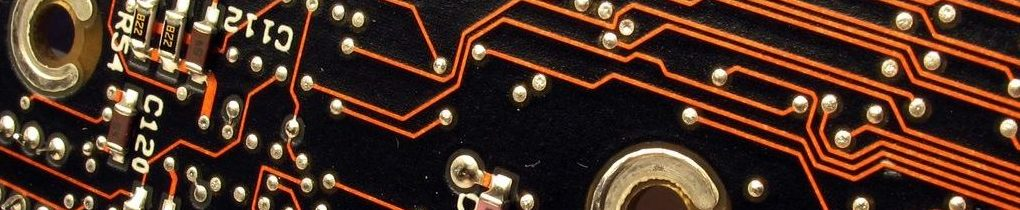# Overview

Her first semester at Great Plains Technology Center Hope was enrolled in digital electronics. She learned how to

• solder
• MultiSim.
• Design circuits using
• Boolean Algebra
• TTL parts
• Programmable Logic Devices.

### Project Lead The Way Digital Electronics Resume

• Computational and Analytical Skills
• Use mathematical processes to convert any value between any number systems
• Calculate voltage, current, and/or resistance for components in a complex circuit
• Translate circuit designs, truth tables, design requirements into logic expressions
• Simplify circuits using Boolean algebra theorems and DeMorgan’s theorems
• Simplify a logic expression graphically using the Karnaugh Mapping process
• Digital Electronics Design Experience
• Implement the design process to design a circuit
• Design a circuit to meet voltage, current, or resistance design requirements
• Select components in a design to produce a desired waveform
• Implement the best combinational logic circuit design
• Apply knowledge of logic gates to select an appropriate gate for the circuit design
• Troubleshoot the design of a circuit by analysis and comparison to the truth table
• Implement a circuit design based on logic expressions
• Troubleshoot existing circuits based on logic expressions
• Determine when NAND only or NOR only implementations are the most efficient
• Implement a seven segment display into a circuit design
• Determine when a common cathode or common anode seven segment display may perform better in a
particular circuit design
• Design a sequential circuit
• Describe the function of XOR/XNOR gates in a circuit design
• Design an adder/subtractor circuit related to the carry out and use on XOR gates
• Design a desired frequency of a clock signal in a 555 timer design
• Design a sequential logic circuit to produce a desired output
• Design synchronous/asynchronous counter circuits based on design requirements
• Design a state machine based on specific design requirements
• Design a circuit with motors as outputs that operate at different voltage levels
• Select and apply the most appropriate design method for circuit implementation
•   Practical Application Experience
• Solder and de-solder components on printed circuit boards
• Validate circuit design through measurement using a probe/oscilloscope and analysis of timing diagram
• Select and apply the most appropriate technology for circuit implementation
• Implement designs on an FPGA
• Create a program to manage inputs and outputs of a microcontroller
•  Tools and Software
• Oscilloscope
• DMM
• FPGA
• Digital Mini System
• Digital Logic Board
•  Professional Skills
• Team collaboration
• Project management
• Problem-solving
• Communication skills
• Presentation skills
• Technical writing
• Course Knowledge
• Foundations of Electronics
• Introduction to safety, electricity, and components
• Introduction to common analog and digital circuit designs and applications
• Combinational Logic
• Designing AOI combinational logic circuits
• Alternative Design: Universal gates and K-mapping
• Specific combinational logic designs
• Introduction to PLD design and circuit prototyping on a PLD
• Sequential Logic
• Sequential logic circuit design
• D flip-flops, J/K flip-flops, and flip-flop applications
• Asynchronous counters
• Counter design SSI, MSI, and MOD asynchronous counters
• Synchronous counters
• Counter design SSI, MSI, and MOD synchronous counters
• Controlling Real World Systems
• Introduction to state machines
• Introduction to sensors, motors, and state machine design
• Introduction to microcontrollers